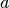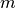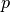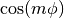# Welcome to PyLaplace!¶

PyLaplace is a Python implementation of generalized Laplace coefficients by three different methods. The generalized Laplace coefficients are defined byThe result is determined by parameters,,,and. These coefficients pop up frequently in celestial mechanics and may need to be evaluated many times, in which case brute force numerical integration is too slow. Pylaplace includes two methods for faster evaluation, one based on hypergeometric functions and an approximate method involving Bessel functions.

## Installation¶

If Python is not installed, download from here and install. The latest versions of Python come with package manager pip included. Then PyLaplace can be installed from the command line simply by entering:

pip install pylaplace


## Usage¶

Within Python, first import the class:

>>> from pylaplace import LaplaceCoefficient


Create an instance of LaplaceCoefficient:

>>> laplace = LaplaceCoefficient()


The generalized Laplace coefficient can then be calculated simply as:

>>> result = laplace(a, s, m, p, q)


The derivative with respect tocan be calculated using:

>>> result = laplace.derivative(a, s, m, p, q)


By default, the Laplace coefficients are calculated using hypergeometric functions. Two other methods are available: ‘Brute’ (brute force integration, very slow) and ‘Bessel’ (fast but approximate). These can be selected at creation by entering:

>>> laplace = LaplaceCoefficient(method='Brute')


# Class reference¶

Class dealing with calculating generalized Laplace coefficients

Generalized Laplace coefficients can be calculated either by brute force integration (slow), hypergeometric functions (faster), or Bessel fuctions (fastest but approximate).

class pylaplace.pylaplace.LaplaceCoefficient(method='Hyper')[source]

Class containing functions to calculate generalized Laplace coefficients.

The generalized Laplace coefficients are defined byParameters: method (str) – way to calculate the coefficients, either ‘Brute’ (brute force integration, slow but exact), ‘Hyper’ (hypergeometric functions, faster and exact), or ‘Bessel’ (Bessel functions, fastest but approximate)
__call__(a, s, m, p, q)[source]

Calculate generalized Laplace coefficient.

Parameters: a (float) – radius-like coordinate, must be smaller than unity s (float) – power to which denominator is raised m (float) – numerator of integrant isp (float) – factor multiplyingin denominator q (float) – constant term in denominator
derivative(a, s, m, p, q)[source]

Calculate derivative with respect toof generalized Laplace coefficient.

Parameters: a (float) – radius-like coordinate, must be smaller than unity s (float) – power to which denominator is raised m (float) – numerator of integrant isp (float) – factor multiplyingin denominator q (float) – constant term in denominator